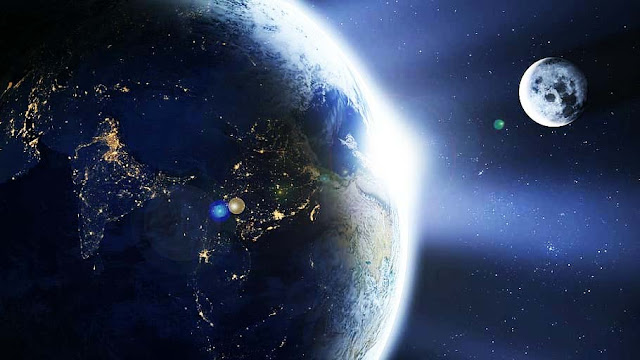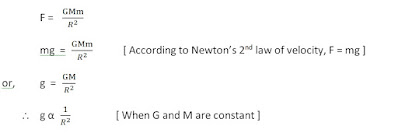# What is Force of gravity? | value of earth and moon gravity

## What is Force of gravity

The force of an object attracting another object to its center is called the force of gravity. The earth pulls any object within its gravitational field towards its center is called the force of gravity of the earth. Like the Earth, other planets and satellites have their own force of gravity. The force of gravity is also called the gravitational force.force of gravity

An object with more mass has more earth's gravity, while an object with less mass has less earth's gravity. The force of gravity applied to an object is also called the weight of the object. Based on Newton's universal law of gravitation, the gravitational force generated on any object on the surface of a planet or satellite can be found.
The force of gravity applied to an object is also called the weight of the object.”
Force of gravity (F) = W = GMm/R2                                           [ Where F = W ]
Here W = force of gravity or weight, M = mass of the planet, m = mass of an object, R = Radius of the planet, and G = Universal Gravitational Constant.
Here the radius of the planet is considered the distance between the planet and the object because there is not much difference between the central distance between the object and the planet and the radius of the planet.

### Force of gravity of the earth

One kilogram of matter on Earth has a gravitational force of 9.8 N.  It can be extracted by this process.
Here,
Mass of earth (M) = 6x1024kg
Radius of earth (R) = 6.38x106m
Mass of object (m) = 1kg
Gravitational constant (G) = 6.67x10-11Nm2kg-2
The force of gravity of earth on object (F) = ?
According to the formula,

So the force of gravity of earth on object (F) =  9.8 N

### Force of gravity of moon

Like the earth, the moon has its own gravitational force. According to the study, the gravitational force of the moon is 1 /6 times less than that of the earth. So a person who jumps up to 2 meters above the surface of the earth can jump up to 12 meters above the surface of the moon.
Here,
Mass of moon (M) = 7.2x1022kg
Radius of moon (R) = 1.7x106kg
Mass of object (m) = 1kg
Gravitational constant (G) = 6.67x10-11Nm2kg-2
The force of gravity of moon on object (F) = ?
According to the formula,

So the force of gravity of the moon on an object (F) = 1.64 N
From the above equation, we can say that the weight of an object is 6 times more on the earth than on the moon.

### Acceleration due to gravity

Gravitational acceleration is the acceleration caused by the gravitational force of any planet. For example, the acceleration caused by the force of gravity on an object falling freely towards the surface of the earth is called the gravitational acceleration of the earth. It’s indicated by ‘g’ and the value of the gravitational acceleration of earth (g) is 9.8m/s2.
The gravitational acceleration that occurs in all objects falling freely in a certain place is the same. That is, the gravitational acceleration that occurs in both large and small objects falling under the same conditions is the same. Here the mass of the body has no effect.
In this regard, the Italian scientist Galileo made an experiment in 1590. In which he dropped large and small objects together from the Leaning Tower of Pisa, both of which reached the ground at the same time.

### The relation between Radius (R) and gravitational acceleration (g)

Suppose M is the mass of a planet, R is the radius and m is the mass of an object on the surface of the planet. According to Newton’s law of gravitation.“The gravitational acceleration generated on a planet or satellite is inversely proportional to the square of its radius.”
Therefore, the gravitational acceleration of an object falling does not affect the mass of the object. And when large or small objects fall in one place, the same acceleration is generated in all of them.

### Acceleration due to gravity of earth

The acceleration due to gravity of the earth is the acceleration that occurs on an object falling freely on the surface of the earth.'g' of earth

The gravitational acceleration generated on any object on Earth is inversely proportional to the square of the radius of Earth. Therefore, the gravitational acceleration is higher in the place where the radius of the earth is less (pole). Gravitational acceleration decreases in places where the radius of the earth is greater (equator).
We know that,

=  9.83 m/s2
Since the earth is not completely spherical, it is compressed in the polar region, so the radius is less and it is inflated in the equatorial region, so the radius is more. So the gravitational acceleration in an object keeps changing according to the location of the earth.
In the polar region g  = 9.83 m/s2
In the equatorial region g = 9.78 m/s2

### Acceleration due to gravity of moon

The mass of the moon is smaller than that of the earth. Therefore, the gravitational force of the moon is less than that of the earth, so that the acceleration due to gravity is also less. The acceleration of gravity on the moon is 1 / 6 times that of the earth. The value of ‘g’ of the moon is 1.67m/s2.

### Different between Mass and Weight

The difference between mass and weight is given below.
 Mass Weight The amount of matter in an object is called mass. The measure of the force of gravity on an object is called weight. The SI unit of mass is kg The SI unit of weight is N (m/s2) It is measured on physical scales. It is measured on spring scales.

According to the formula,
W = mg
Or,          W α g                   [Where ‘m’ is constant]
It means weight always depends on acceleration due to gravity.
The value of 'G' varies in different parts of the earth so the weight of the same mass also varies according to the location.

I sincerely hope that I have given you a lot of information about what is force of gravity, as well as its unit, formula, and the value of earth, and moon gravity, etc. Please comment if you have any confusion. I request all of you readers to share this information on various social media so that our awareness remains and it will benefit everyone. I need your help so that I can give you more new information. Please subscribe to our website.
Thank you!